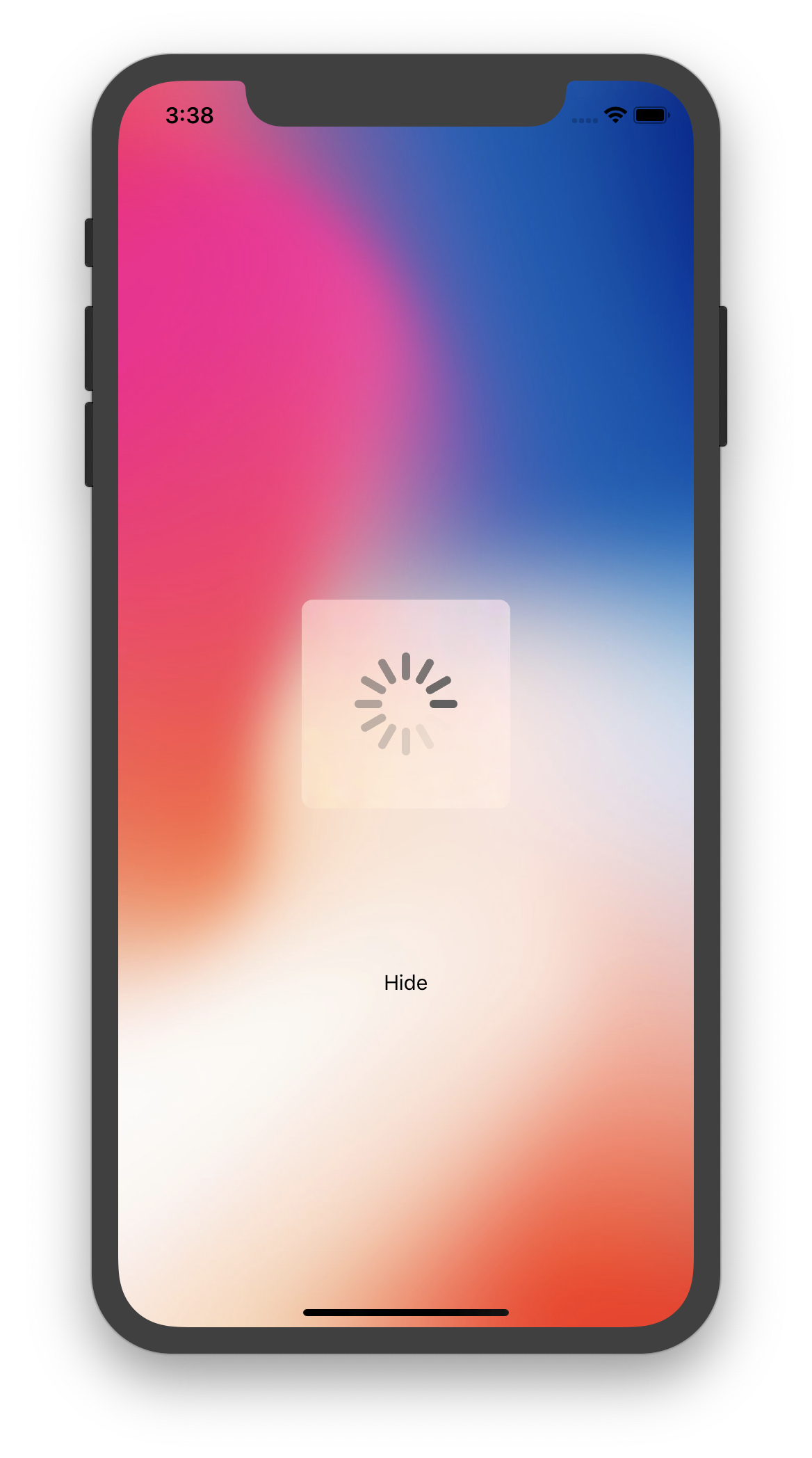Issue #230

CAReplicatorLayer is a layer that creates a specified number of sublayer copies with varying geometric, temporal, and color transformations

Here we use `instanceTransform` which applies transformation matrix around the center of the replicator layer

Below is how we use `replicatorLayer` to replicate lots of `line` and rotate them around the center.

``````let replicatorLayer = CAReplicatorLayer()
let animation = CABasicAnimation(keyPath: #keyPath(CALayer.opacity))

let line = CALayer()
let lineCount: Int = 12
let duration: TimeInterval = 1.0
let lineSize: CGSize = CGSize(width: 20, height: 6)
let lineColor: UIColor = UIColor.darkGray

let angle = CGFloat.pi * 2 / CGFloat(lineCount)
let rotation = CATransform3DMakeRotation(angle, 0, 0, 1.0)

replicatorLayer.instanceTransform = rotation
replicatorLayer.instanceCount = lineCount
replicatorLayer.instanceDelay = duration / TimeInterval(lineCount)

line.backgroundColor = lineColor.cgColor
line.frame.size = lineSize

animation.fromValue = 1.0
animation.toValue = 0.0
animation.repeatCount = Float.greatestFiniteMagnitude
animation.timingFunction = CAMediaTimingFunction(name: .linear)
animation.duration = duration

Pay attention to `position` of the `line`. The larger the `x`, the closer to center. `y` should be half the height of the replicator layer size, changing it affects the skewness of the `line`.